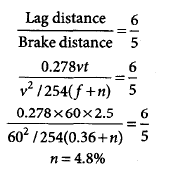Courses

# Test: Transportation Engineering- 1

## 15 Questions MCQ Test GATE Civil Engineering (CE) 2022 Mock Test Series | Test: Transportation Engineering- 1

Description
This mock test of Test: Transportation Engineering- 1 for GATE helps you for every GATE entrance exam. This contains 15 Multiple Choice Questions for GATE Test: Transportation Engineering- 1 (mcq) to study with solutions a complete question bank. The solved questions answers in this Test: Transportation Engineering- 1 quiz give you a good mix of easy questions and tough questions. GATE students definitely take this Test: Transportation Engineering- 1 exercise for a better result in the exam. You can find other Test: Transportation Engineering- 1 extra questions, long questions & short questions for GATE on EduRev as well by searching above.
QUESTION: 1

### If the load, warping and frictional stresses in a cement concrete slab are 210 N/mm2 , 90 N/mm2 and 10N/mm2 respectively. The critical combination of stresses during summer mid-day is

Solution:

For Summer mid-day ,

S = S + Sw - Sf = 210 + 90 - 10 = 290 N/mm2

QUESTION: 2

### The superelevation needed for a vehicle travelling at a speed of 60 km/h on a curve of radius 128 m a surface with a coefficient of friction of 0.15 is

Solution: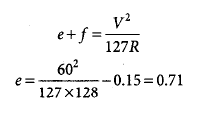QUESTION: 3

### The maximum super elevation to be provided on a road curve is 1 in 15. If the rate of change of super elevation is specified as 1 in 120 and the road width is 10m, then the minimum length of the transition curve on the either end will be

Solution:

Provide an assumption in the question  Assuming super-elevation introduction with respect to inner edge.

Since only superelevation (e = 1/15), width of road(W=10m) and rate of super-elevation (N=120) is provided. Therefore, using one of the formula to determine the length of transition curve (Ls) when super-elevation is introduced with respect to inner edge.

L_s=ⅇNW = 1/15×120×10 = 80 m

QUESTION: 4

In highway geometric design, once the cumulative speed distribution is drawn, the design adequacy is checked at

Solution:

In cumulative speed distribution following values must be designed for:
- 98th percentile speed: check for design adequacy
- 85th percentile speed: Upper speed limit
- 15th percentile speed: Lower speed limit

QUESTION: 5

Penetration to know the grade of bitumen is measured in

Solution:

Penetration value is the vertical distance traversed by the point of a standard needle into the bituminous material under specific conditions of load, time and temperature.
The distance is measured in one tenths of a millimetre.
It mean a penetration value of 80 means the actual penetration is 8mm.

QUESTION: 6

For total reaction time of 2.5 s, coefficient of friction 0.35, and design speed of 80 km/h, what is the stopping sight distance on a highway?

Solution: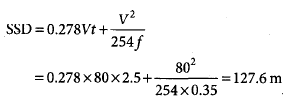QUESTION: 7

What is the superelevation for a horizontal highway curve of radius 500 m and speed 100 km/h in mixed traffic condition?

Solution: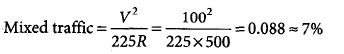QUESTION: 8

The length of a transition curve for a circulation curve of radius 300 m and for a design speed of 15 m/s, when the rate of change of centrifugal acceleration is 0.3 m/s3 is

Solution: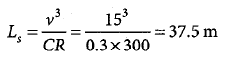QUESTION: 9

Bitumen grade 80/100 indicates that under the standard test conditions, penetration value of bitumen would vary from

Solution: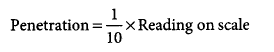*Answer can only contain numeric values
QUESTION: 10

Modulus of subgrade reaction using 30 cm diameter plate is obtained as 200 N/cm3. The value of the same (in N/cm3) using the standard plate will be __________ N/cm3.

Solution: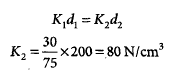*Answer can only contain numeric values
QUESTION: 11

A rest vertical curve joints two gradients of +3% and –2% for a design speed of 80 km/h and the corresponding stopping sight distance of 120 m. The height of driver’s eye and the object above the road surface are 1.20 m and 0.15 m, respectively. The curve length (which is less than stopping sight distance) to be provided is _________ m.

Solution: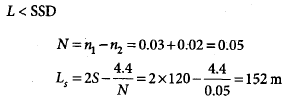QUESTION: 12

The design speed for a two-lane road is 80 km/h. When a design vehicle with a wheel base of 6.6 m is negotiating a horizontal curve on that road, the off-tracking is measured as 0.096 m, the required widening of carriageway of the two-lane road on the curve is approximately

Solution: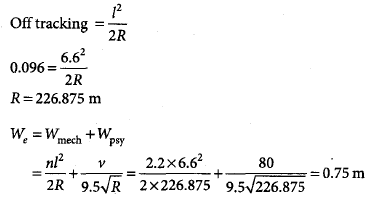QUESTION: 13

It is proposed to widen and strengthen an existing two lane NH section as a divided highway. The existing traffic in one direction is 2500 commercial vehicles (CV) per day. The construction will take 1 yr.

The design CBR of soil subgrade is found to be 5% Given: Traffic growth rate for CV = 8% Vehicle damage factor = 3.5 (standard axles per CV) Design life = 10 yr, Traffic distribution factor = 0.75

The cumulative standard axles 9 (msa) computed are

Solution: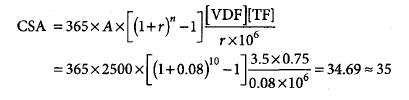QUESTION: 14

The width of the expansion joint is 20 m in a cement concrete pavement. The laying temperature is 20° C and the maximum slab temperature in summer is 60° C. The coefficient of thermal expansion of concrete is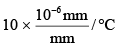and the joint filler compresses upto 50% of the thickness. The spacing between expansion joints should be

Solution: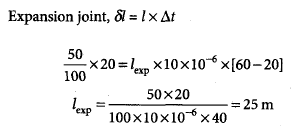QUESTION: 15

A vehicle moving at 60 km/h on an ascending gradient of a highway has to come to stop position to avoid collision with a stationary object. The ratio of lag to brake distance is 6:5. Considering total reaction time of the driver as 2.5 s and the coefficient of longitudinal fraction as 0.36, the value of ascending gradient (%) is

Solution: